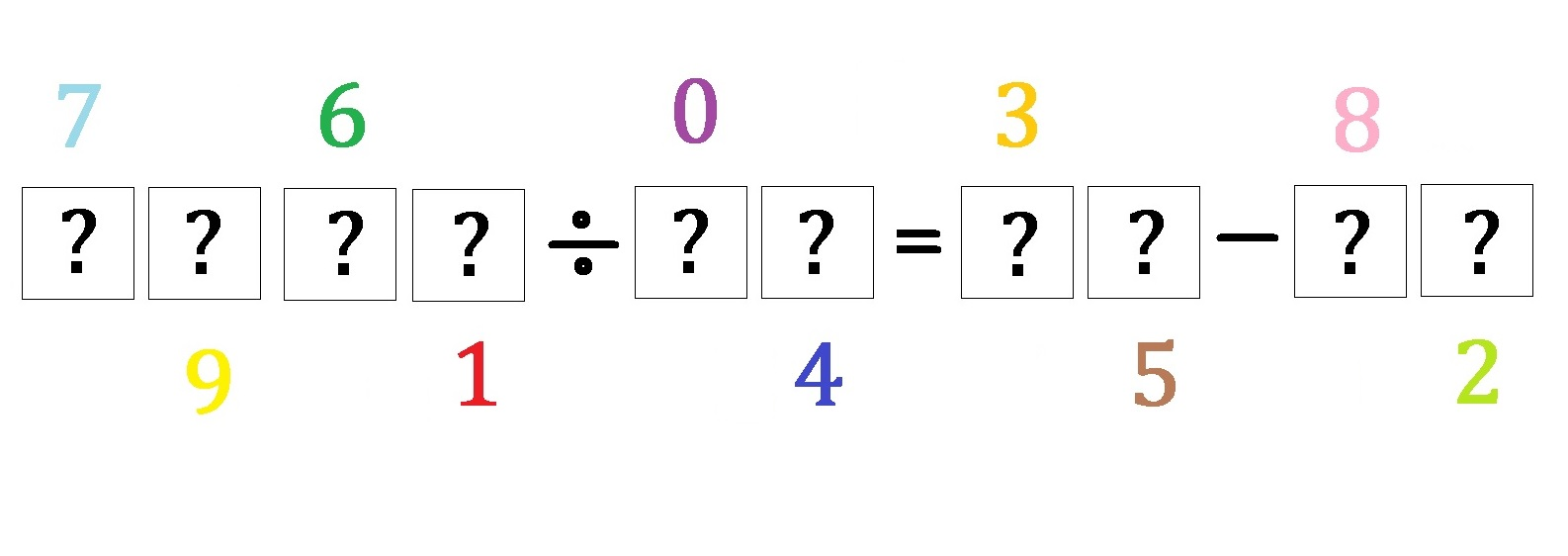# Can you fill in these boxes? (Part II)Within the boxes above, each contains a distinct digit from 0 to 9 such that the quotient, with a prime divisor, on the left equals to the difference on the right and that the sum of the four digits of the numerator equals to the sum of two digits of the divisor.

What is the value of this equation?

×

Problem Loading...

Note Loading...

Set Loading...